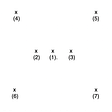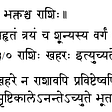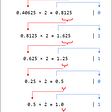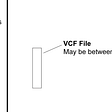# Solutions to Systems of Linear Equations using python

In this article, we will discuss how we solve a systems of equations when it has unique solution. We will discuss some of the common methods that you often come across in your work in this section. And in the next section, we will show you how to solve it in Python.

Consider a system of linear equations in matrix form, AX=y, where A is an n×n matrix. Recall that this means there are nequations and n unknowns in our system. A solution to a system of linear equations is an x in Real numbers system that satisfies the matrix form equation.

Let’s say we have n equations with n variables, AX=y, as shown in the following:

There are some famous methods could be found in every elementary linear algebra book,such as Gauss Elimination Method, Gauss-Jordan Elimination Method, LU Decomposition Method and Iterative Methods — Gauss-Seidel Method.But in this article we do not investigate these methods,consequently therefore we focus on numerical analysis programming.

Now we will use Python to solve the systems of equations. The easiest way to get a solution is via the solve function in Numpy.

Let’s try numpy.linalg.solve to solve the following equations:

`import numpy as npA = np.array([[4, 3, -5],               [-2, -4, 5],               [8, 8, 0]])y = np.array([2, 5, -3])x = np.linalg.solve(A, y)print(x)`

The result will be :

`[ 2.20833333 -2.58333333 -0.18333333]`

The solver is actually doing a LU decomposition to get the results. You can check the help of the function, it needs the input matrix to be square and of full-rank, i.e., all rows (or, equivalently, columns) must be linearly independent.

Now let’s try to solve the above equations using the matrix inversion approach.

`A_inv = np.linalg.inv(A)x = np.dot(A_inv, y)print(x)`

The result will be :

`[ 2.20833333 -2.58333333 -0.18333333]`

We can also get the L and U matrices used in the LU decomposition using the scipy package.

`from scipy.linalg import luP, L, U = lu(A)print('P:\n', P)print('L:\n', L)print('U:\n', U)print('LU:\n',np.dot(L, U))`

The result will be :

`P: [[0. 0. 1.] [0. 1. 0.] [1. 0. 0.]]L: [[ 1.    0.    0.  ] [-0.25  1.    0.  ] [ 0.5   0.5   1.  ]]U: [[ 8.   8.   0. ] [ 0.  -2.   5. ] [ 0.   0.  -7.5]]LU: [[ 8.  8.  0.] [-2. -4.  5.] [ 4.  3. -5.]]`

Programming skills which were reviewed above are useful in scientific computing solving many problems in engineering.In the next articles we will follow up these methods in the field of finance trying to build quantitative algorithms.

We wish to escalate public knowledge about computer science topics.NetCoinCapital is a blockchain start up which is motivated to expand the bounds of science by trending,researching and developing state of art technologies for future concepts.

--

--

--

## More from Netcoincapital Official

Does small or a big role matter? Anyone who puts all his energy into his position will benefit by reaching the goal. https://linktr.ee/socialmediasNCC

Love podcasts or audiobooks? Learn on the go with our new app.

## Powering Through that Math Degree —  What’s Next?## Liberal Arts Blog — The Orion of Math — Counting, Formulating, Calculating## 3 Ways to solve a Quadratic equation (3rd is my favorite) -## A game called Numbers Four Fours## Who defined Division by Zero as Infinity?## Netcoincapital Official

Does small or a big role matter? Anyone who puts all his energy into his position will benefit by reaching the goal. https://linktr.ee/socialmediasNCC

## Analysing my Magic: The Gathering Commander Deck using Monte Carlo Simulations with Python## Convert a Negative Decimal Number with a Fractional Part using 2’s Complement in Python## Fetching subsets of VCF files, a DIY approach